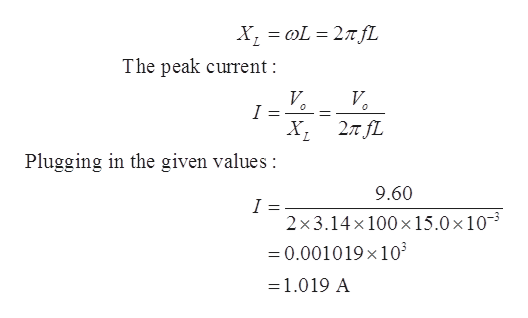# A 15.0 mH inductor is connected across an AC generator that produces a peak voltage of 9.60 V.a. What is the peak current through the inductor if the emf frequency is 100 Hz?b. What is the peak current through the inductor if the emf frequency is 100 kHz?

Question
12 views

A 15.0 mH inductor is connected across an AC generator that produces a peak voltage of 9.60 V.

a. What is the peak current through the inductor if the emf frequency is 100 Hz?

b. What is the peak current through the inductor if the emf frequency is 100 kHz?

check_circle

Step 1

Given values:

Inductance,     L = 15.0 mH = 15.0 x 10-3 H

Peak voltage Vo = 9.60 Hz

Step 2

(a)

Frequency, f = 100 Hz

Inductive reactance:

...help_outlineImage TranscriptioncloseX L2 fL The peak current: V I 2л /L Plugging in the given values : 9.60 = 2 x3.14x 100 x 15.0 x 10 =0.001019 x 103 1.019 A fullscreen

### Want to see the full answer?

See Solution

#### Want to see this answer and more?

Solutions are written by subject experts who are available 24/7. Questions are typically answered within 1 hour.*

See Solution
*Response times may vary by subject and question.
Tagged in

### Other